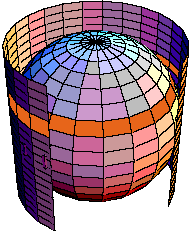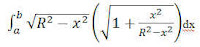Monday, 18 October 2010

Surprisingly Constant

Some things never change... and some of the things that don’t change are pretty remarkable. I was recently reminded of two that always seemed non-intuitive, and therefore quite pleasing. Both of these can be proved toward the end of a first year calculus course, and at least one can be explained with a little hand-waving, to a good pre-calc student.

The first is the simple fact that if you take a slice a sphere with a pair of parallel planes that are some distance, d, apart; the surface area of the part of the sphere between those planes is the same no matter where the slices are made. Near the “poles” or near the “equator”, the area is the same. It is nice to be able to tell students that this incredibly interesting fact was known to Archimedes in the first Century of the modern era when he proved that the surface area of a sphere is the same as the lateral area of a cylinder that contains the sphere.This image is part of an explanation provided at a math site at the University of Regina

The essence of the explanation uses nothing beyond similar triangles. If we approximate the sphere’s surface between the slicing planes as a frustum of a cone, then the slant height (AP) can be shown to be in the same proportion to the height (AB)as the radius of the frustum (QP) is to the radius of the sphere(KP)).This image is also from the U Regina site mentioned above.Another nice thing about this relation is that when you set up the rather fearsome looking integral for the surface area, it reduces through simple algebra to a constant.The second similarly interesting idea is about the volume of a shape I call a bead, for lack of a more precise term. The bead is the solid remaining when you drill through the center of a sphere.The unexpected constant here is that for any radius sphere you start with, the volume of the bead depends only on the height. And perhaps even less expected, is that the volume is the same as the volume of an un-drilled sphere with the same height (i.e., when 2r = h).

I have not found a simple explanation for pre-calc students that will explain this one. If you have one in your pocket, drop me a note.

Arjen Dijksman said...

Interesting!

For the bead's volume, the way I see it is to use Mamikon's annular sweep. The volume of the bead is the sum of the annular slice areas πa² where a changes linearly from 0 to h/2 and back to 0, which is the the same as for the volume of a sphere of radius h/2.

Pat B said...

You lost me for the moment... I have to go back and re-read the blog on Mamikon's idea... But thanks for a great approach... I keep thinking it takes calculus, and it just isn't always so..

Arjen Dijksman said...

Yes, you're right, I need to draw it. I'll try to come up with a picture later on...

Miguel said...

That's right. You have to show that the area of a horizontal section at a distance d from the equator is independent of the radius of the sphere.

When you write this out you find the difference of two differences of squares. The radius of the sphere cancels out and you're left with (h/2)^2 - d^2